Question

# Please asnwer all the question 1) Your portfolio consists of \$3,000 in ABC stock, \$4,500 of...

1) Your portfolio consists of \$3,000 in ABC stock, \$4,500 of DEF stock and \$2,500 of GHI stock. Expected rates of return are ABC 5%, DEF 12%, and GHI 16%. What is the portfolio expected rate of return? A) 10.9%
B) 12.0%
C) 11.4%
D) 16.0%

2) You are considering investing in a portfolio consisting of 40% Electric General and 60% Buckstar. If the expected rate of return on Electric General is 16% and the expected return on Buckstar is 9%, what is the expected return on the portfolio?
A) 12.50%
B) 13.20% C) 11.80%
D) 10.00%

3) The expected return on VZ next year is 12% with a standard deviation of 20%. The expected return on ANT next year is 24% with a standard deviation of 30%. The correlation between the two stocks is .6. If Emily makes equal investments in VZ and ANT, what is the standard deviation of her portfolio?
A) 22.47%
B) 25.00%
C) 5.05%
D) 15.00%

4) The expected return on ZV next year is 12% with a standard deviation of 20%. The expected return on TNA next year is 24% with a standard deviation of 30%. The correlation between the two stocks is -.6. If Hannah makes equal investments in ZV and TNA, what is the standard deviation of her portfolio?
A) 22.47%.
B) 12.04%
C) 1.45%.
D) 16.00%.

5) What is the expected dollar return on a portfolio which consists of \$9,000 invested in an S&P 500 Index fund, \$32,500 in a technology fund, and \$8,500 in Treasury Bills. The expected rate of return is 11% on the S&P Index fund, 14% on the technology fund and 2% on the Treasury Bills.
A) \$13,640
B) \$571
C) \$4,500 D) \$5,710

6) Your broker mailed you your year-end statement. You have \$25,000 invested in Amazon, \$18,000 tied up in Boeing, \$36,000 in Caterpillar stock, and \$11,000 in DuPont. The betas for each of your stocks are 1.43 for Amazon, .79 for Boeing, 1.37 for Caterpillar, and 1.71 for DuPont. What is the beta of your portfolio?
A) 1.33 B) 1.31
C) 1.00
D) 5.30

7) On average, when the overall market changes by 10%, the stock of Veracity Communications changes 12%. Veracity's beta is
A) 1.2.
B) 8.33%.
C) 12%.
D) Insufficient information is provided

8) Bell Weather, Inc. has a beta of 1.25. The return on the market portfolio is 12.5%, and the risk-free rate is 5%. According to CAPM, what is the required return on this stock?
A) 20.62%
B) 9.37%
C) 14.38%
D) 15.62%

9) The expected return on the market portfolio is currently 11%. Battmobile Corporation stockholders require a rate of return of 23.0%, and the stock has a beta of 2.5. According to CAPM, determine the risk-free rate.
A) 17.5%
B) 2.75%
C) 3.0%
D) 9.2%

Dear student, only one question is allowed at a time. I am answering the first question

1)

Expected return of a portfolio is the weighted average of returns of the stocks of the portfolio

 Calculations A B = A / Total A C D = B x C Particulars Investment Percentage of Investment Expected Return Weighted Average return ABC 3000 30% 5 1.50 DEF 4500 45% 12 5.40 GHI 2500 25% 16 4.00 Total 10000 10.90

So, as per above calculations, option A is the correct option

#### Earn Coins

Coins can be redeemed for fabulous gifts.

Similar Homework Help Questions
• ### QUESTION 54 Your portfolio consists of \$50,000 invested in Stock X and \$50,000 invested in Stock ...please assist with excel function or calculator QUESTION 54 Your portfolio consists of \$50,000 invested in Stock X and \$50,000 invested in Stock Y. Both stocks have return of 15%, betas of 1.6, and standard deviations of 30%-The returns ofthe two stocks are independent correlation coefficient between them, xy, is zero. Which of the following statements best describes the cl your 2-stock portfolio? Your portfolio has a beta greater than 1.6, and its expected return is greater than 15% Your...

• ### Question 1: Cooley Company's stock has a beta (b) of 1.28, the risk-free rate (rRF) is...

Question 1: Cooley Company's stock has a beta (b) of 1.28, the risk-free rate (rRF) is 1.25%, and the market risk premium (RPM) is 5.50%. a. What does the beta measure? Give a short answer in 1 sentence. b. What is market risk premium? Give a short answer in 1 sentence. c. Calculate the firm's required rate of return? Show the step-by-step calculation and circle your answer. (Hint: Required return = rRF + b(RPM)) Question 2: Consider the following information...

• ### Question 1: Cooley Company's stock has a beta (b) of 1.28, the risk-free rate (rRF) is...

Question 1: Cooley Company's stock has a beta (b) of 1.28, the risk-free rate (rRF) is 1.25%, and the market risk premium (RPM) is 5.50%. a. What does the beta measure? Give a short answer in 1 sentence. b. What is market risk premium? Give a short answer in 1 sentence. c. Calculate the firm's required rate of return? Show the step-by-step calculation and circle your answer. (Hint: Required return = rRF + b(RPM)) Question 2: Consider the following information...

• ### P.14 An investor holding a portfolio consisting of two stocks invests 25% of assets in Stock...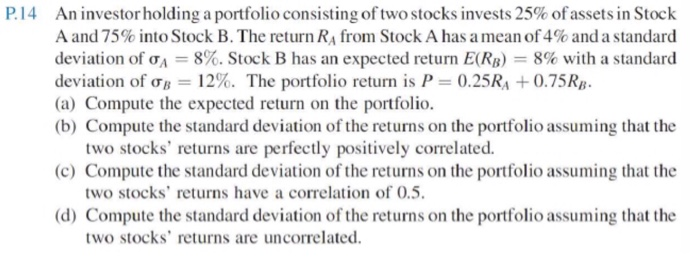P.14 An investor holding a portfolio consisting of two stocks invests 25% of assets in Stock A and 75% into Stock B. The return RA from Stock A has a mean of 4% and a standard deviation of A = 8%. Stock B has an expected return E(RB) = 8% with a standard deviation of ob = 12%. The portfolio return is P = 0.25RA +0.75RB. (a) Compute the expected return on the portfolio. (b) Compute the standard deviation of...

• ### Suppose Intel stock has a beta of 1.64, whereas Boeing stock has a beta of 0.96....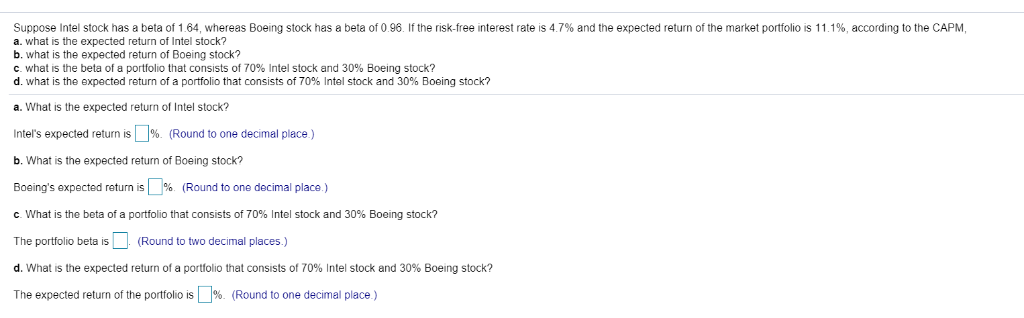Suppose Intel stock has a beta of 1.64, whereas Boeing stock has a beta of 0.96. If the risk-free interest rate is 4.7% and the expected return of the market portfolio is 11.1%, according to the CAPM, a. what is the expected return of Intel stock? b. what is the expected return of Boeing stock? c. what is the beta of a portfolio that consists of 70% Intel stock and 30% Boeing stock? d. what is the expected return of...

• ### : Question Help P 12-32 (similar to) Suppose Intel stock has a beta of 1.64, whereas...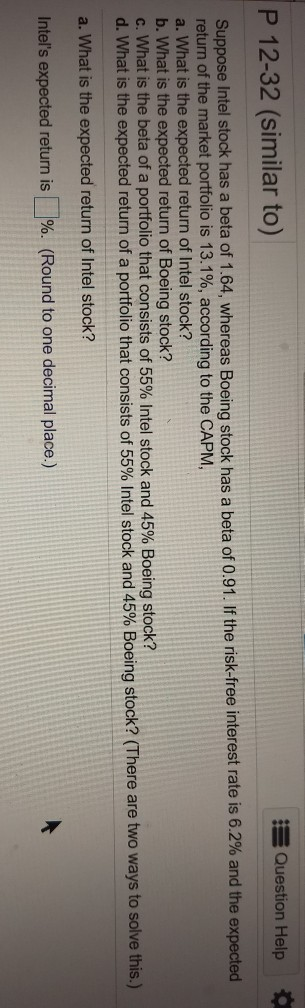: Question Help P 12-32 (similar to) Suppose Intel stock has a beta of 1.64, whereas Boeing stock has a beta of 0.91. If the risk-free interest rate is 6.2% and the expected return of the market portfolio is 13.1%, according to the CAPM, a. What is the expected return of Intel stock? b. What is the expected return of Boeing stock? c. What is the beta of a portfolio that consists of 55% Intel stock and 45% Boeing stock?...

• ### There are three stocks in the market, stock A, stock B, and stock C. The price...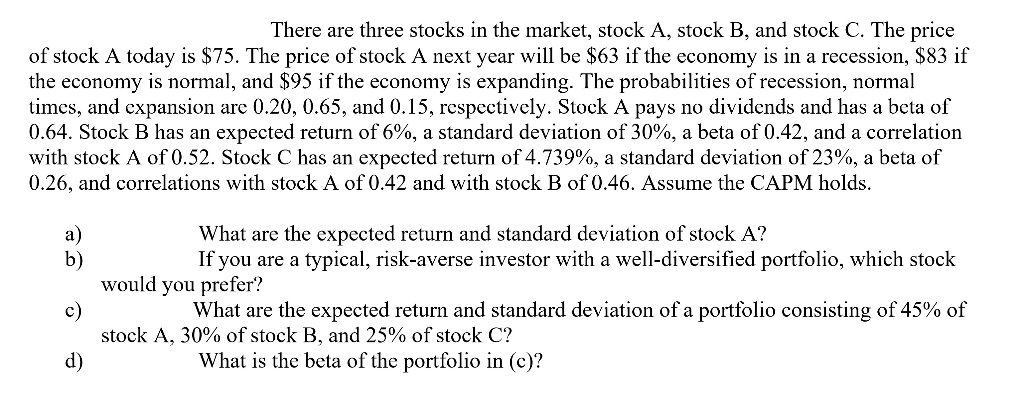There are three stocks in the market, stock A, stock B, and stock C. The price of stock A today is \$75. The price of stock A next year will be \$63 if the economy is in a recession, \$83 if the economy is normal, and \$95 if the economy is expanding. The probabilities of recession, normal times, and expansion are 0.20, 0.65, and 0.15, respectively. Stock A pays no dividends and has a beta of 0.64. Stock B has...

• ### Please show all work. Thanks! An optimal risky portfolio has been developed with investments in stocks...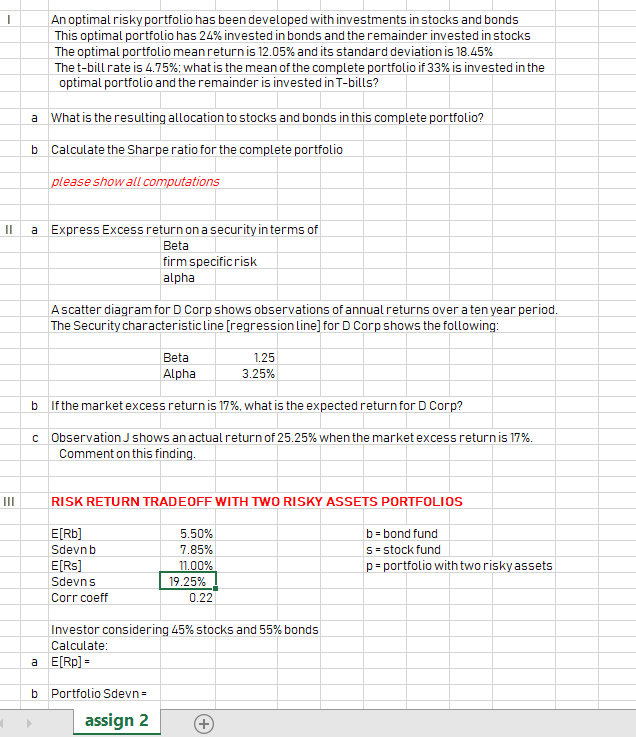Please show all work. Thanks! An optimal risky portfolio has been developed with investments in stocks and bonds This optimal portfolio has 24% invested in bonds and the remainder invested in stocks The optimal portfolio mean return is 12.05% and its standard deviation is 18.45% The t-bill rate is 4.75%; what is the mean of the complete portfolio if 33% is invested in the optimal portfolio and theremainder is invested in T-bills? a What is the resulting allocation to stocks...

• ### P 12-32 (similar to) Suppose Intel stock has a beta of 1.46, whereas Boeing stock has...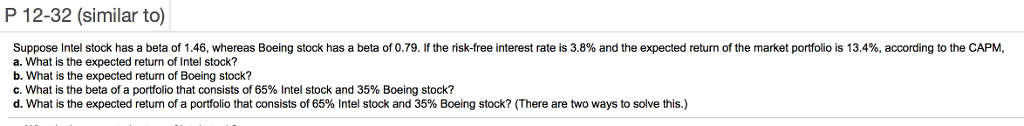P 12-32 (similar to) Suppose Intel stock has a beta of 1.46, whereas Boeing stock has a beta of 0.79. If the risk free interest rate is 3.8% and the expected return of the market portfolio is 13.4%, according to the CAPM a. What is the expected return of Intel stock? b. What is the expected retum of Boeing stock? C. What is the beta of a portfolio that consists of 65% Intel stock and 35% Boeing stock? d. What...

• ### Consider the following information on a portfolio of three stocks: State of Probability of Stock A...

Consider the following information on a portfolio of three stocks: State of Probability of Stock A Stock B Stock C Economy State of Economy Rate of Return Rate of Return Rate of Return Boom .14 .11 .36 .51 Normal .51 .19 .21 .29 Bust .35 .20 − .20 − .39 a. If your portfolio is invested 44 percent each in A and B and 12 percent in C, what is the portfolio’s expected return, the variance, and the standard deviation?...